• ## matlab矩阵卷积

热门讨论 2011-07-17 19:15:30
这是一个实现矩阵卷积计算的matlab源代码，经实验可用。
• Matlab应用(这里考虑二维矩阵，在图像中对应)个图像模糊（边缘）操作，假设矩阵A、B，A代表源图像，B代表卷积模板，那么B的取值决定最后运算的结果。  Matlab中的应用函数——conv2（二维卷积，一维对应conv）...

Matlab应用(这里考虑二维矩阵，在图像中对应)两个图像模糊（边缘）操作，假设矩阵A、B，A代表源图像，B代表卷积模板，那么B的取值决定最后运算的结果。

Matlab中的应用函数——conv2（二维卷积，一维对应conv）

函数给出的公式定义为：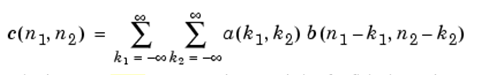同一维数据卷积一样，它的实质在于将卷积模板图像翻转（旋转180），这里等同于一维信号的翻转，然后将卷积模板依次从上到下、从左到右滑动，计算在模板与原始图像交集元素的乘积和，该和就作为卷积以后的数值。

为了验证后续矩阵卷积转化为矩阵相乘，这里给出的conv2的实例描述：

假设矩阵A（4*3）、B（2*3）如下：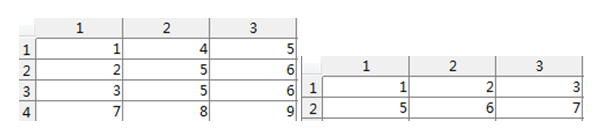首先，B需要旋转180，

命令旋转2次90即可：

B = rot90(rot90(B));或者B = rot90(h,2);  结果为：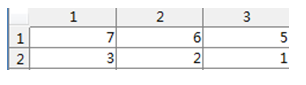其次：命令conv2函数：

C = conv2(A,B，‘shape’),该函数的具体操作图示：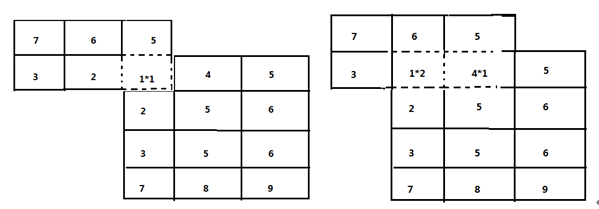依次计算直至结束，结果数据为：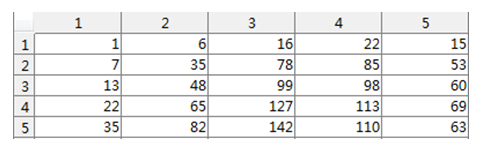shape的取值有三种，full代表返回卷积以后的全部数据，size为(mA+mB-1,nA+nB-1)的数据；same代表返回卷积以后的原图size (mA,nA)的部分数据；valid返回size为(mA-mB+1,nA-nB+1)的数据，指的是模板元素全部参加运算的结果数据，即源图像和模板的交集为模板。

展开全文• 二维矩阵卷积个图像大小相同 二维矩阵卷积个图像大小相同
• 之前图形学课上老师在 Image Filters中讲过用矩阵卷积对图像进行处理，当时一直不懂卷积是怎么个东东，今天... 矩阵卷积转化为矩阵相乘形式——Matlab应用(这里考虑二维矩阵，在图像中对应)个图像模糊（边缘）操
之前图形学课上老师在 Image Filters中讲过用矩阵卷积对图像进行处理，当时一直不懂卷积是怎么个东东，今天网上找到下面的博客，恍然大悟。

转自：http://blog.csdn.net/anan1205/article/details/12313593

两个矩阵卷积转化为矩阵相乘形式——Matlab应用(这里考虑二维矩阵，在图像中对应)两个图像模糊（边缘）操作，假设矩阵A、B，A代表源图像，B代表卷积模板，那么B的取值决定最后运算的结果。

Matlab中的应用函数——conv2（二维卷积，一维对应conv）

函数给出的公式定义为：同一维数据卷积一样，它的实质在于将卷积模板图像翻转（旋转180），这里等同于一维信号的翻转，然后将卷积模板依次从上到下、从左到右滑动，计算在模板与原始图像交集元素的乘积和，该和就作为卷积以后的数值。

为了验证后续矩阵卷积转化为矩阵相乘，这里给出的conv2的实例描述：

假设矩阵A（4*3）、B（2*3）如下：首先，B需要旋转180，

命令旋转2次90即可：

B = rot90(rot90(B));或者B = rot90(h,2);  结果为：其次：命令conv2函数：

C = conv2(A,B，‘shape’),该函数的具体操作图示：依次计算直至结束，结果数据为：shape的取值有三种，full代表返回卷积以后的全部数据，size为(mA+mB-1,nA+nB-1)的数据；same代表返回卷积以后的原图size (mA,nA)的部分数据；valid返回size为(mA-mB+1,nA-nB+1)的数据，指的是模板元素全部参加运算的结果数据，即源图像和模板的交集为模板。

矩阵卷积转化为矩阵相乘，网上也有很多方法，通俗化表示为：

A×B = B1*A1;

需要针对原始数据与模板数据做变换，变换过程如下：首先进行周期延拓，补零：

M = mA+mB-1 = 5;  N = nA+nB-1 = 5，对应卷积以后full数据大小。

那么初次换换的A和B为：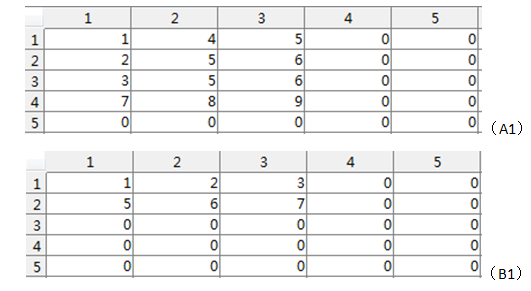其次对A1和B1分别进行变换

转化B1——针对B1以及转换矩阵方法为：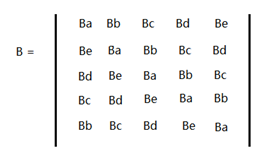将B1中的每一行向量依次按照B转化为一个方形矩阵Ba~Be，然后针对于每一个方形矩阵按照B矩阵组合成一个新的矩阵B1。B1矩阵的大小为（（mA+mB-1）*（nA+nB-1），（mA+mB-1）*（nA+nB-1））。

转化A1——堆叠向量式

将上个步骤转换的A1按照行向量顺寻依次转化为一个列向量，那么列向量的大小为（（mA+mB-1）*（nA+nB-1），1）大小。针对实例：具体代码为：

周期延拓：

转化A——>A1

[plain] view
plaincopy

[m1,n1] = size(A);  [m2,n2] = size(B);
m=m1+m2-1;n=n1+n2-1;
AA =AA';
A1 = AA(:);%%%%

转化B——>B1

[plain] view
plaincopy

B2(1,:) = BB(1,:);
for i =2:m
B2(i,:) = BB(m-i+2,:);
end %%%矩阵a ~ e的重新赋值

B4 = zeros(n,n);%%%%%%%每一行转化的方阵
B1 = zeros(m*n,m*n);%%%%%最后的矩阵
for i =1:m%%%%%%%%几维向量
B = B2(i,:);
if sum(sum(abs(B))==0)
B4 = zeros(n,n);
else
for j = 1:n%%%%%%%元素
for k =0:n-1%%%%%%%%位置(搞定一行向量转化为方阵的形式)
t = mod(j+k,n);
if t==0
t = n;
end  %%%end if
B4(t,k+1) = B(j);
end %%%end for
end  %%%end for
for  k =0:m-1%%%%%%%%每一个转换矩阵在大矩阵中的位置编号（搞定小方阵在大阵中的位置转化为大方阵的形式）
t = mod(i+k,m);
if t==0
t = m;
end  %%%end if
B1(k*n+1:(k+1)*n,(t-1)*n+1:t*n) = B4;
end  %%%end for
end %%%end if else
end  %%%end for

结果数据转化：

[plain] view
plaincopy

Result = B1*A1;
Result = reshape(Result,n,m);
Result = Result';

得到的结果等同于conv2的数据结果：展开全文• 矩阵卷积原理与实现 function [ hp] = juanji( f,g ) %此函数只为计算任意二维矩阵的卷积 %命令格式：C=juanji(A,B) C为A B的卷积; %若A为m*n矩阵，B为p*q矩阵，则C为(m+p-1)*(n+q-1)矩阵;
• ## verilog实现矩阵卷积运算

千次阅读 多人点赞 2019-05-24 09:23:19
它提供了个数组相乘的方式，个数组拥有不同的大小，但是具有相同的维数，生成了一个用于相同维数的新数组。可以用来图像的执行操作，输入一组特定的像素值线性组合成为另一组像素值。在图像处理中常见的msk运算...
verilog实现卷积运算
卷积的运算原理
卷积是一种线性运算，是很多普通图像处理操作的基本算法之一。它提供了两个数组相乘的方式，两个数组拥有不同的大小，但是具有相同的维数，生成了一个用于相同维数的新数组。可以用来图像的执行操作，输入一组特定的像素值线性组合成为另一组像素值。在图像处理中常见的msk运算都是卷积，广泛应用于图像滤波。
1.1卷积出现的背景
卷积是在信号与线性系统的基础或背景中出现的，脱离这个背景单独谈卷积是没有任何意义的，除了那个所谓褶反公式上的数学意义和积分（或求和，离散情况下）。
信号与线性系统，讨论的就是一个信号经过一个线性系统以后发生的变化（就是输入、输出和所经过的所谓系统，这三者间的运算关系）。
因此，实际都是要根据我们需要处理的信号形式，来设计所谓的系统传递函数，那么这个系统的传递函数和输入信号，在数学上的形式就是所谓的卷积关系。
卷积关系最重要的一种情况，就是在信号与线性系统或数字信号处理中的卷积定理。利用该定理，可以将时间域或空间域中的卷积运算等价为频率域的相乘运算，从而利用FFT等快速算法，实现有效的计算，节省运算代价。卷积的本质是滑动平均思想，它就是一种微元相乘累加的极限形式。卷积本身不过是一种数学运算而已，就跟“蝶形运算”一样，在信号与系统中，y(t)=f(t)*h(t)。时域的卷积等于频域的乘积，即有Y(s)=F(s)×H(s)，拉氏变换后得到的函数其实就是信号的频域表达式）。
然而，在通信系统中，我们关心的以及要研究的是信号的频域，不是时域，原因是因为信号的频率是携带有信息的量。所以，我们需要的是这个表达式，但是实际上，我们往往不能很容易得到。
卷积其实就是通过两个函数 f和g生成第三个函数的一种数学算法，表征函数与经过翻转和平移的的重叠面积。如果将参加卷积的一个函数看作区间的指示函数，卷积也可以被看做是“移动平均”的推广。
图1.1中两个方形脉冲波做卷积。其中函数首先对反射，接着平移“t”,成为。那么重叠部分的面积就相当于“”处的卷积，其中横坐标代表待积变量以及新函数的自变量。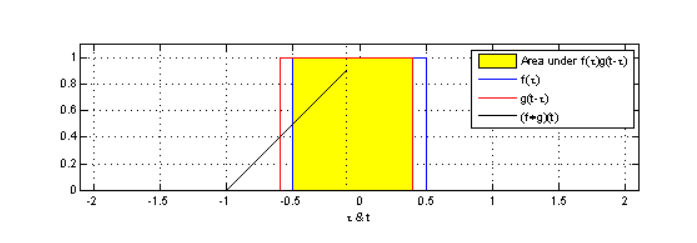构造一个3 * 3的卷积核，并利用该卷积核完成与6×6矩阵的卷积运算，数据位宽8bit补码数, 结果位宽20bit补码数。
卷积的基本过程如下：
对卷积核进行180度翻转（数据读写顺序的调度）将3×3卷积核的中心对准 6×6 矩阵的每个数进行对应数据乘累加得出结果，如此往复作业。输入数据补码8bit，实际有效7bit，输出数据补码20bit，实际有效19bit，卷积增加位数3×3=9，所以单个乘法最多增加19-7-9=3bit。所以卷积核采用3bit数，即4bit补码数。
内置电路图如图二所示：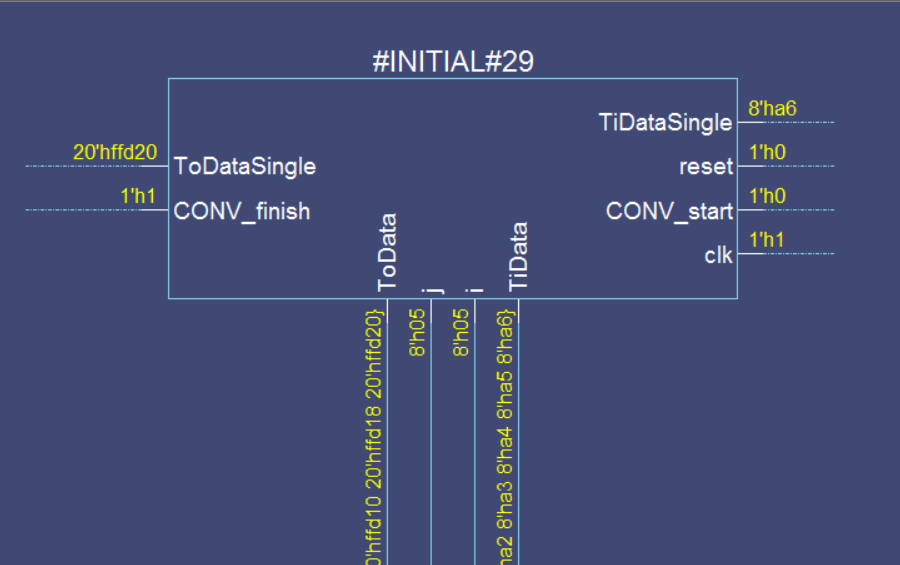正如第二部分对本次设计的介绍，我们要做到的是对模拟信号的采样由A/D转换器来完成，而卷积过程由信号的移位来实现。为了设计卷积运算器，首先要设计RAM 和A/D转换器的VerilogHDL 模型。在电子工业发达的国家，可以通过商业渠道得到非常准确的外围器件的虚拟模型。如果没有外围器件的虚拟模型。因为RAM和A/D转换器不是我们设计的硬件对象，所以需要的只是他们的行为模型，精确的行为模型需要认真细致的编写，并不比综合模块容易编写。
运算过程简介
系统内置3×3的4bit补码数的卷积核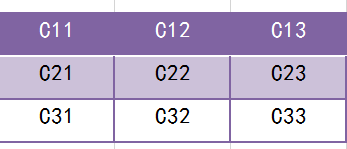外部输入6*6的8比特补码数：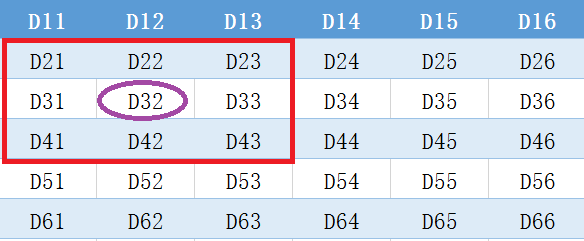工作过程：系统将反转后的卷积核与上图某一紫色圈圈数据和其周围的红色框框中的数据进行相乘并相加。直到所有红色矩形框中的部分均进行过卷积操作。
举例如下，对应第一个要进行卷积操作的数D32
输出数据为：O32= D21C33 + D22C32 + D23C31+ D31C23 + D32C22 + D33C21+ D11C13 + D42C12 + D43*C11 ；
工作说明
每次启动后TB读取要卷积的数据，并将此数据传输给CONV，每次传输一个数据即8bit。
CONV接收完数据后开始卷积。卷积结束后把数据传输给TB，每次传输一个数据即20bit。
结果验证
Python中的Scipy包致力于科学计算中常见问题的各个工具箱。它的不同子模块相应于不同的应用。例如：插值、积分、优化、图像处理、统计、特殊函数等等。通常用于计算numpy矩阵，有效便捷。
1）Python中卷积实现的原理：对于in_c个通道的输入图，如果需要经过卷积后输出out_c个通道图，那么总共需要in_cout_c个卷积核参与运算。例如，输入为[h:5,w:5,c:4]，那么对应输出的每个通道，需要4个卷积核。输出为3个通道,所以总共需要34=12个卷积核。对于单个输出通道中的每个点，取值为对应的一组4个不同的卷积核经过卷积计算后的和。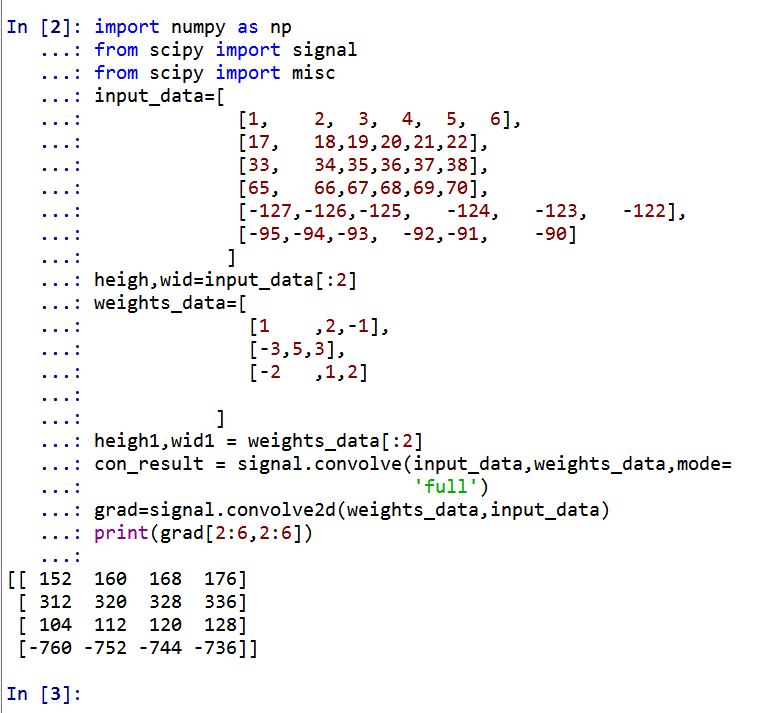仿真说明
Modelsim可以支持命令行的方式，通过创建do文件，可以集成多个可执行的命令。那么对于前期一边编写代码，一边进行功能仿真，使用do文件是可以明显提高工作的效率。
编写wave文件对其进行波形仿真：在仿真前Transcript中命令：do wave.do加载预设波形；输出结果在Transcript中查看，输出结果如图：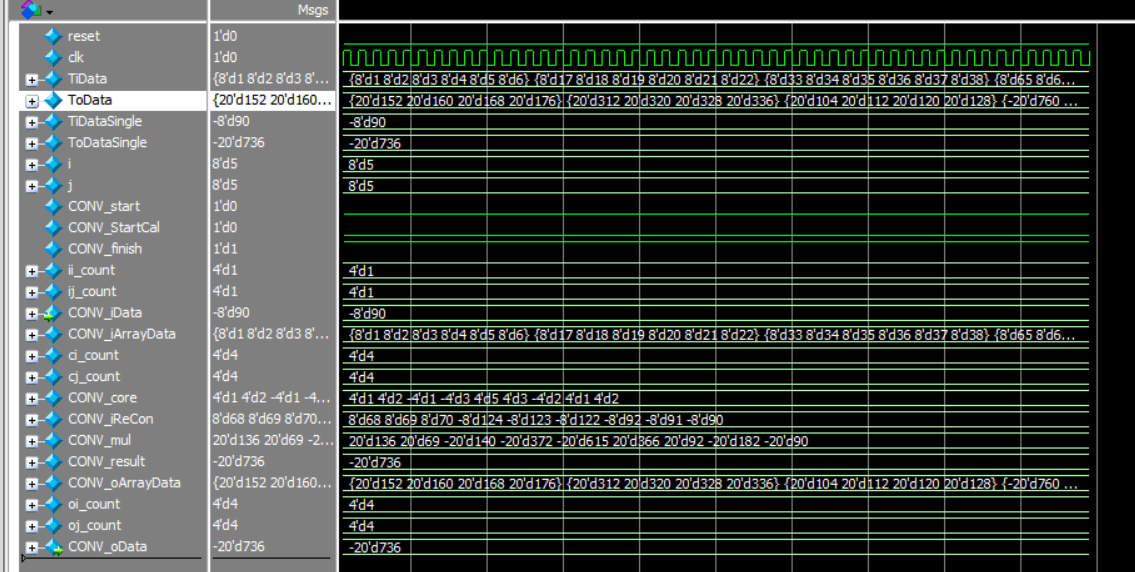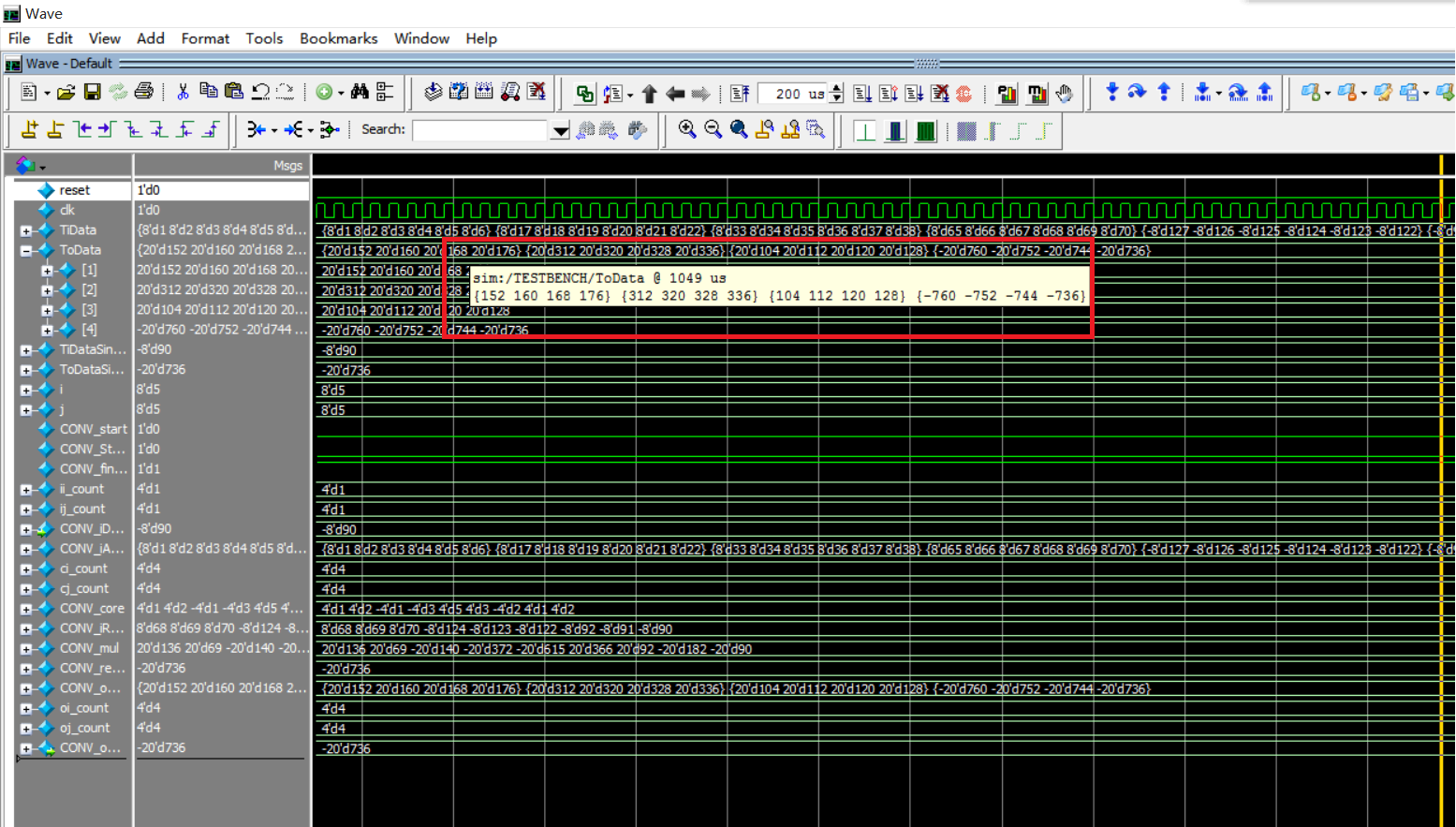可以看到上图的红色框框中的输出结果与python的验证结果相同，仿真结果图中的输入数据与input_data中的数据一致。
我们以输入为6个通道的矩阵作为输入、3*3的卷积核、1个通道宽高分别为4的输出，作为结果验证。
在modelsim，我们主要对testbench进行仿真。testbench代码如下：
//TESTBENCH
timescale 1us/1us
module TESTBENCH();
reg  signed  [7:0] TiData[1:6][1:6];  // Test input  Data
reg  signed [19:0] ToData[1:4][1:4];  // Test output Data
reg  signed  [7:0] TiDataSingle;  // for transmission
wire signed [19:0] ToDataSingle;  // for transmission
reg clk;
reg reset;
reg CONV_start;
wire CONV_finish;
reg [7:0] i;
reg [7:0] j;
parameter period = 10;
parameter hperiod = 5;
CONV CONV_T(
.reset(reset),
.clk(clk),
.CONV_start(CONV_start),
.CONV_finish(CONV_finish),
.CONV_iData(TiDataSingle),
.CONV_oData(ToDataSingle));
initial
begin
$display("0.Load Data");$readmemh("Data_input.txt", TiData);
for(i = 1; i < 7; i = i + 1)
display("%d %d %d %d %d %d", TiData[i], TiData[i], TiData[i], TiData[i], TiData[i], TiData[i]); clk = 0; CONV_start = 0; reset = 1; // Reset Chip #period reset = 0; // Chip Working #period CONV_start = 1; // CONV start and writing data // align test data to the negedge of clkdisplay("1.Write Data");
for(i = 1; i < 7; i = i + 1)
for(j = 1; j < 7; j = j + 1)
begin
TiDataSingle = TiData[i][j];
#period;
end
CONV_start = 0; // finish writing data
$display("2.Convolution"); while(!CONV_finish) #period; #period;$display("3.Read  Data");
for(i = 1; i < 5; i = i + 1)
for(j = 1; j < 5; j = j + 1)
begin
ToData[i][j] = ToDataSingle;
end
for(i = 1; i < 5; i = i + 1)
$display("%d %d %d %d", ToData[i], ToData[i], ToData[i], ToData[i]);$display("End")：
end
always #hperiod clk = !clk;
endmodule


verlog源码
module CONV(
input wire reset,
input wire clk,
input wire CONV_start,
output reg CONV_finish,
input wire signed  [7:0] CONV_iData,
output reg signed [19:0] CONV_oData
);

reg signed [3:0]CONV_core[1:9];

reg  [3:0] ii_count;
reg  [3:0] ij_count;
reg  [3:0] ci_count;
reg  [3:0] cj_count;
reg  [3:0] oi_count;
reg  [3:0] oj_count;

reg  signed  [7:0] CONV_iArrayData[1:6][1:6];  // input  Data
reg  signed [19:0] CONV_oArrayData[1:4][1:4];  // output Data
reg  CONV_StartCal;  // Start convolution

// For ReConstruct
wire signed  [7:0] CONV_iReCon[1:9];  // input ReConstruct Temp
wire signed [19:0] CONV_mul[1:9];
wire signed [19:0] CONV_result;

// Calculating Convolution
assign CONV_iReCon = CONV_iArrayData[ci_count+0][cj_count+0];
assign CONV_iReCon = CONV_iArrayData[ci_count+0][cj_count+1];
assign CONV_iReCon = CONV_iArrayData[ci_count+0][cj_count+2];
assign CONV_iReCon = CONV_iArrayData[ci_count+1][cj_count+0];
assign CONV_iReCon = CONV_iArrayData[ci_count+1][cj_count+1];
assign CONV_iReCon = CONV_iArrayData[ci_count+1][cj_count+2];
assign CONV_iReCon = CONV_iArrayData[ci_count+2][cj_count+0];
assign CONV_iReCon = CONV_iArrayData[ci_count+2][cj_count+1];
assign CONV_iReCon = CONV_iArrayData[ci_count+2][cj_count+2];

assign CONV_mul = CONV_core*CONV_iReCon;
assign CONV_mul = CONV_core*CONV_iReCon;
assign CONV_mul = CONV_core*CONV_iReCon;
assign CONV_mul = CONV_core*CONV_iReCon;
assign CONV_mul = CONV_core*CONV_iReCon;
assign CONV_mul = CONV_core*CONV_iReCon;
assign CONV_mul = CONV_core*CONV_iReCon;
assign CONV_mul = CONV_core*CONV_iReCon;
assign CONV_mul = CONV_core*CONV_iReCon;

assign CONV_result = CONV_mul + CONV_mul + CONV_mul +
CONV_mul + CONV_mul + CONV_mul +
CONV_mul + CONV_mul + CONV_mul;

// Init Core
always @(posedge reset)
begin
CONV_core <= 4'h1;
CONV_core <= 4'h2;
CONV_core <= 4'hf;
CONV_core <= 4'hd;
CONV_core <= 4'h5;
CONV_core <= 4'h3;
CONV_core <= 4'he;
CONV_core <= 4'h1;
CONV_core <= 4'h2;
end

always @(posedge clk or posedge reset or posedge CONV_finish)
begin
if(reset || CONV_finish)
begin
ii_count <= 1;
ij_count <= 1;
CONV_StartCal <= 0;
end
else if(CONV_start && (ii_count < 7))
begin
if(ij_count < 6)  ij_count <= ij_count + 1;
else
begin
if(ii_count < 6)begin ii_count <= ii_count + 1; ij_count <= 1;  end
else            begin CONV_StartCal <= 1; end
end
CONV_iArrayData[ii_count][ij_count] <= CONV_iData;  // Load Data
end
end

// Convolution
always @(posedge clk or posedge reset)
begin
if(reset)
begin
ci_count <= 1;
cj_count <= 1;
CONV_finish <= 0;

end
else if(CONV_StartCal && (ci_count < 5))
begin
if(cj_count < 4)            cj_count <= cj_count + 1;
else
begin
if(ci_count < 4)  begin ci_count <= ci_count + 1; cj_count <= 1;  end
else              begin CONV_finish <= 1; end
end

CONV_oArrayData[ci_count][cj_count] <= CONV_result; // Record the Result
end
end

// Output Data
always @(posedge clk or posedge reset or posedge CONV_start)
begin
if(reset || CONV_start)
begin
oi_count <= 1;
oj_count <= 1;
end
else if(CONV_finish && (oi_count < 5))
begin
if(oj_count < 4)  oj_count <= oj_count + 1;
else
begin
if(oi_count < 4)begin oi_count <= oi_count + 1; oj_count <= 1;  end

end
CONV_oData <= CONV_oArrayData[oi_count][oj_count];  // Output Data
end

end

endmodule

python验证
import numpy as np
from scipy import signal
from scipy import misc
input_data=[
[1,	2,	3,	4,	5,	6],
[17,	18,19,20,21,22],
[33,	34,35,36,37,38],
[65,	66,67,68,69,70],
[-127,-126,-125,	-124,	-123,	-122],
[-95,-94,-93,	-92,-91,	-90]
]
heigh,wid=input_data[:2]
weights_data=[
[1	,2,-1],
[-3,5,3],
[-2	,1,2]

]
heigh1,wid1 = weights_data[:2]
con_result = signal.convolve(input_data,weights_data,mode=
'full')

小编还在成长，请大家多多指教！


展开全文• 矩阵A与矩阵B，其中矩阵B为卷积模板，B1是卷积模板翻转180度，FA是矩阵A在频域下的矩阵，FB是矩阵B在频域下的矩阵。 结论：矩阵A与矩阵B1相乘=矩阵A与矩阵B的卷积=矩阵FA与矩阵FB相乘。 （PS：上面说的个相乘是...
通过查阅了网上很多的资料，在此做一个总结，有错误之处，还请评论指出，谢谢！
设矩阵A与矩阵B，其中矩阵B为卷积模板，B1是卷积模板翻转180度，FA是矩阵A在频域下的矩阵，FB是矩阵B在频域下的矩阵。
结论：矩阵A与矩阵B1相乘=矩阵A与矩阵B的卷积=矩阵FA与矩阵FB相乘。
（PS：上面说的两个相乘是不同的，具体可以从下面的讲述中可以清楚的明白）
1）验证：矩阵A与矩阵B1相乘=矩阵A与矩阵B的卷积
假设矩阵A为5×5的矩阵，矩阵B为3×3的矩阵。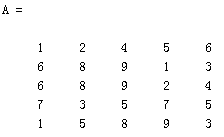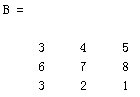B1是B旋转180度的矩阵，B1=rot90(rot90(B))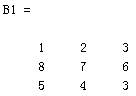矩阵A与矩阵B1的相乘过程如下图所示：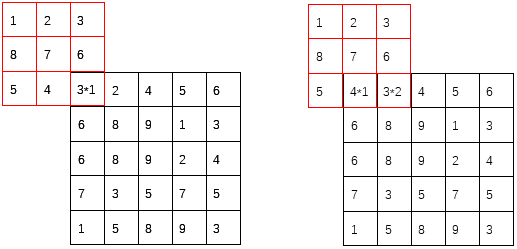依次计算，最后的结果为：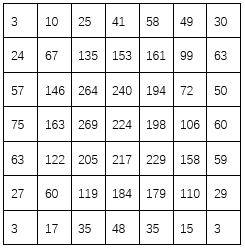通过matlab来证明conv2(A,B)与上述操作的结果一致：
（关于im2col函数的作用可以参考:https://blog.csdn.net/dwyane12138/article/details/78449898）
为了让im2col()函数更明显，可以输出A2。
A=[1 2 4 5 6;6 8 9 1 3;6 8 9 2 4;7 3 5 7 5;1 5 8 9 3];%5*5
B=[3 4 5;6 7 8;3 2 1];%卷积核 3*3
C1=conv2(A,B,'full') %直接用卷积函数conv2的卷积结果
A2=im2col(A1,[3 3]);%块数据向量化
B1=B(:);%变成一个列向量
B2=B1(end:-1:1);%倒序
B2=B2';%转置
C2=reshape(B2*A2,[7 7])%卷积转换为乘积并且转化为结果数据

结果如下图所示：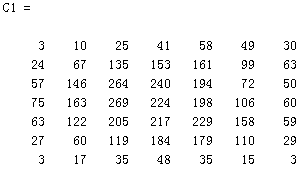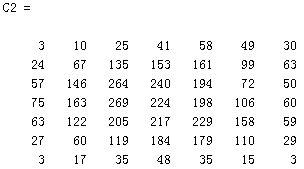运算结果成功证明：矩阵A与矩阵B1相乘=矩阵A与矩阵B的卷积。
2）证明：矩阵A与矩阵B的卷积=矩阵FA与矩阵FB相乘
A=[1 2 4 5 6;6 8 9 1 3;6 8 9 2 4;7 3 5 7 5;1 5 8 9 3];%5*5
B=[3 4 5;6 7 8;3 2 1];%卷积核 3*3
C1=conv2(A,B,'full') %直接用卷积函数conv2的卷积结果
FA=fft2(A,7,7);
FB=fft2(B,7,7);
%FC=FA.*FB;  频域乘积，结果就是下面的for循环的操作，从这里可以看出，上面提到的两个相乘的方法是不同的。
for i=1:5
for j=1:5
FC(i,j)=FA(i,j)*FB(i,j);
end
end
C3=ifft2(FC); %频域转换

结果入下图所示：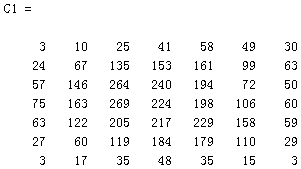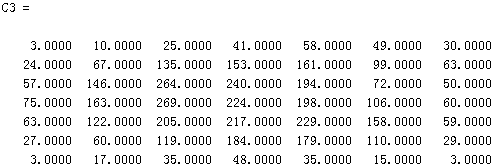运算结果成功证明：矩阵A与矩阵B的卷积=矩阵FA与矩阵FB相乘。
综上所述：矩阵A与矩阵B1相乘=矩阵A与矩阵B的卷积=矩阵FA与矩阵FB相乘。

`
展开全文• 此程序要求输入矩阵矩阵A任意形状即可，只要大于矩阵B即可， 输入要求： 第一行： 输入个整数，代表矩阵A的行数和列数 接下来输入n行，即输入矩阵A 接下来输入矩阵B（卷积核的高） 接下输入m行 Eg: 3 ...笔试真题
• 题目一个M×N的矩阵A，以及一个K×K的矩阵B，对矩阵卷积运算，复杂度为多少？有没有快速运算？思路如果考虑暴力法，那复杂度显然是O(MNK2)O(MNK^2)。快速算法当时一直在往动态规划上套，死活想不出来，%>_<%
• 1 5 7] %b矩阵如多数模板一样取3*3那么conv(a,b)的结果肯定是（5+3-1）*（4+3-1）=7*6的矩阵卷积计算过程如下：默认先把a矩阵补0变成7*6维的矩阵，然后b翻转 之后进行模板操作，要计算a矩阵中哪个点卷积...
• % 时卷频乘，可用于求矩阵卷积 [ra,ca] = size(A); [rb,cb] = size(B); r = ra+rb-1; % A，B个矩阵做卷积后其行数和列数分别为A，B矩阵的行列数相加减1 c = ca+cb-1; a1 = [A zeros(ra,c-ca);zeros(r-ra,c)]; % ...matlab function fft
• 转载自：http://blog.csdn.net/anan1205/article/details/12313593矩阵卷积转化为矩阵相乘形式——Matlab应用(这里考虑二维矩阵，在图像中对应)个图像模糊(边缘)操作，假设矩阵A、B，A代表源图像，B代表卷积...
• 矩阵矩阵的二维卷积 矩阵与数组的卷积 数组与数组的卷积 数组与矩阵卷积
• 2.对矩阵进行卷积则是矩阵点乘后加和的结果。 卷积神经网络 1 . 顾名思义就是加入卷积层的神经网络算法（除此以外还有一个池化层，后续会继续讲解） 2.卷积神经网络的好处在于参数共享，自动提取特征以及平移...python 算法 大数据
• import numpy as np f = np.array([[1,2,1], [1,0,0], [-1,0,1]]) # f = np.array([[3,4,4], # [1,0,2], # [-1,0,3]]) img = np.array([ [2,3,7,4,6,2,9], [6,6,9,8,7,4,3], [3,4,8,3,8,9,...
• 本文关注数字信号的卷积、反卷积基本概念的理解与矩阵实现卷积LTI（线性时不变）系统常用卷积来表征。卷积的定义为： 这个描述加上公式，如同一记闷棍，直接把很多初学者打翻在地。类似的形式很多教材都在用，我当年...
• 卷积将为每个5x5补丁计算32个特征 .现在让我们接受这个，并且稍后问自己为什么有32个功能以及为什么有5x5补丁 .它的重量张量将具有[5,5,1,32]的形状 . 前个维度是补丁大小，下一个是输入通道的数量，最后一个是...
• 看到篇论文，分别用宽卷积和窄卷积对句子进行卷积，记录一下。一篇是Severyn A, Moschitti A. Modeling Relational Information in Question-Answer Pairs with Convolutional Neural Networks[J]. 2016（论文稍微...
• 本文关注数字信号的卷积、反卷积基本概念的理解与矩阵实现卷积LTI（线性时不变）系统常用卷积来表征。卷积的定义为： 这个描述加上公式，如同一记闷棍，直接把很多初学者打翻在地。类似的形式很多教材都在用，我当年...
• conv2是计算矩阵的二维卷积1、MATLAB中提供了卷积运算的函数命令conv2，其语法格式为：C = conv2(A,B)C = conv2(A,B)返回矩阵A和B的二维卷积C。若A为ma×na的矩阵，B为mb×nb的矩阵，则C的大小为(ma+mb+1)×(na+...
• 相关和卷积个相反的概念，卷积需要将模板矩阵旋转180°,当然可以相对地将原图像和模板重合部分做一个旋转，简化计算。 下面是C代码实现分别用相关和卷积实现滤波。 #include&lt;stdio.h&gt; #include...
• 分别使用FFT和矩阵乘法实现线性卷积，并在CPU和GPU种情况下比较运行时间。
• 简单来讲，卷积是一种函数和函数产生一个新函数的数学运算，该数学运算的自变量是个函数f, g（连续或离散都可以,，定义域之外的部分记函数值填充为0），输出为一个函数h,满足 ,或者说，就是对每个自变量t, 的h(t)...原理
• 一维卷积卷积定义对于个长度分别为m和n的序列x(i)和g(i)有， h(i)=x(i)∗g(i)=∑jx(j)g(i−j) h(i)=x(i)*g(i)=\sum_{j}x(j)g(i-j) 上式给出了长度为N=m+n-1的输出序列。称为一维情况下的卷积公式。循环矩阵参考...图像处理...